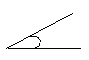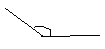Email us to get an instant 20% discount on highly effective K-12 Math & English kwizNET Programs!

#### Online Quiz (WorksheetABCD)

Questions Per Quiz = 2 4 6 8 10

### Grade 4 - Mathematics2.3 Types of Angles

 When two rays meet at a point called vertex an angle is formed.An angle that measures 90 degrees is called right angle.An angle that measures less than 90 degrees is called acute angle.An angle that measures more than 90 degrees is called obtuse angle.Directions: Answer the following questions. Also draw right angle, acute angle, obtuse angle and name the rays, angles and vertex.
 Q 1: The angle WXY is an acute angle.FalseTrue Q 2: What do you call an angle that forms a square corner?right angleobtuse anglesquare angle Q 3: When two rays have the same endpoint, they form what?linean anglesquare Q 4: An angle that is greater than a right angle is calleda great angleobtuse angleacute angle Question 5: This question is available to subscribers only! Question 6: This question is available to subscribers only!